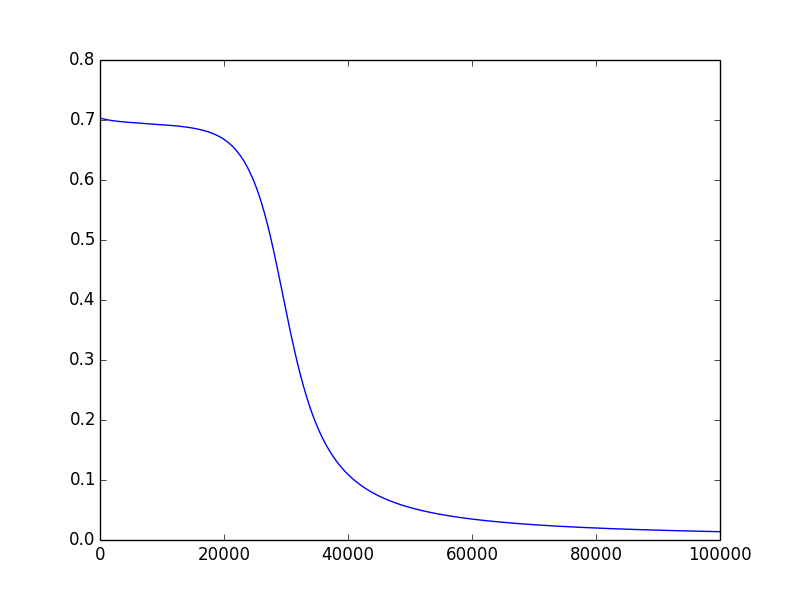I have been meaning to refresh my memory about neural networks. As any beginner would do, I started with the XOR problem. In fact, this was the first neural network problem I solved when I was in grad school.

### XOR truth table

For the uninitiated, the XOR truth table looks as follows:

Input1 Input2 Output
0 0 0
0 1 1
1 0 1
1 0 1

This is in fact how a two-way switch works. If both the switches are on or off, then the output is off. If only one is on, then the output is on.

### Python implementation

First, let us import all the Python packages needed to implement this neural network. We are going to implement a neural network with two layers (one hidden and one output). As an exercise, you can try to implement this logic with a single layer with a single neuron (it’s not possible ;) )

For the activation functions, let us try and use the sigmoid function for the hidden layer. For the output layer, we don’t have a choice. We have to use a sigmoid since we want our outputs to be 0 or 1. A sigmoid output followed by a threshold operation should do it.

### Initialization of the neural network parameters

This step is very important and is very easy to mess up. For example, if you initialize all the weights to zero, your network won’t learn anything. In our case, we are initializing all the weights to random numbers between 0 and 1. Bias terms are initialized to 0. Instead of passing around multiple variables, we are using a dictionary to pass all the weights and biases.

### Forward Propagation

Forward propagation is very simple to implement. It just involves multiplication of weights with inputs, addition with biases and sigmoid function. Note that we are storing all the intermediate results in a cache. This is needed for the gradient computation in the back propagation step. Also, we compute the cost function just like we do for logistic regression but only over all the samples.

### Backward Propagation

This is the most complicated of all the steps in designing a neural network. I am not going to go over all the details of the implementation but just give an intuition. I recommend you take the Machine Learning on Coursera to get a much better understanding.

Intuition behind back-prop is that we want to make small changes to the weights and biases so that our network output moves towards the expected output. This is done by the differentiating the cost function with respect to the weights and moving in the direction of the negative of the gradient (since we want to minimize the cost function). To calculate the gradients, we use the cache values from the forward propagation.

### Updating the weights

As we said before, we are updating the weights based on the negative gradients.

### Putting it all together

Using all the methods we discussed, we are going to put together the complete neural network model to learn the XOR truth table. First, we define the inputs and outputs as 2D arrays. Next, we compute the number of input features (2), number of output features (1) and set the number of hidden layer neurons. The beauty of this code is that you can reuse it for any input/output combinations as long as you shape the X and Y values correctly.

Each column of X (Y) holds an input (output). Within a column, we have all the features as rows. Next, we call the initialize_parameters function to initialize the weights. Next, we have two hyperparameters - num_iterations and learning_rate. These two need to be carefully chosen for your problem. For example, choosing a large learning rate may cause the network to diverge but a small learning rate may take forever to converge. Next, we create an array for storing the cost function (losses) at the end of every iteration.

It is just magic after that. Do a forward propagation and compute the intermediate results cache. Follow it up with a back-prop which spits out the gradients. Finally, update the parameters using the computed gradients. Rinse and repeat.

### Evaluating the performance

How do we test the network? We just need to do forward propagation again with the learned parameters. To compute the output, we can use 0.5 as a threshold. Finally, we are also plotting the losses to see how the cost function varied with each iteration. If everything is right, cost function should continuously decrease.

In one of the runs, my network produced the following values for the inputs X (which perfectly models the XOR function).

[[ 0.00148372  0.998589    0.99887018  0.00154541]]
[[ 0.  1.  1.  0.]]


### Cost function### Where to go from here?

Feel free to play around with the training algorithm to model other logic gates. For example, here are the Y values for a few logic gates. See if you can model all of them (you should be able to).

### A few gotchas

I have made a lot of design choices here without saying how we came up with those numbers. Number of hidden layer neurons, Number of iterations, Learning rate etc., As I mentioned earlier, these are called hyperparameters and are usually decided by using a validation set. In our case, since we are just memorizing these values (and the data set is so tiny), we don’t have that luxury. So, I just tried several nominal values until one worked. When I tried the same code on a Windows machine, the network failed to converge several times but on a Mac it always converged. I believe it has to do with the initialization randomness. So, if it does not converge for you the first time, try a few times and see if it works (before hunting for a bug).